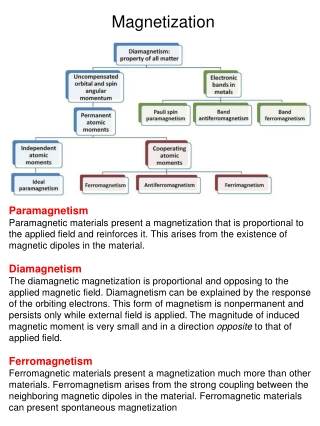DownloadDownload PresentationMagnetization

# Magnetization

Télécharger la présentation## Magnetization

- - - - - - - - - - - - - - - - - - - - - - - - - - - E N D - - - - - - - - - - - - - - - - - - - - - - - - - - -
##### Presentation Transcript

1. Magnetization Paramagnetism Paramagnetic materials present a magnetization that is proportional to the applied field and reinforces it. This arises from the existence of magnetic dipoles in the material. Diamagnetism The diamagnetic magnetization is proportional and opposing to the applied magnetic field. Diamagnetism can be explained by the response of the orbiting electrons. This form of magnetism is nonpermanent and persists only while external field is applied. The magnitude of induced magnetic moment is very small and in a direction opposite to that of applied field. Ferromagnetism Ferromagnetic materials present a magnetization much more than other materials. Ferromagnetism arises from the strong coupling between the neighboring magnetic dipoles in the material. Ferromagnetic materials can present spontaneous magnetization

2. Torque on the magnetic dipole The torque tries to line the dipole up parallel to the field If the field is uniform, the net force is zero: If the field is not uniform, the net force is:

3. Magnetic moments of electrons Consider the Bohr model of the atom, where electrons travel in circular orbits around the nucleus. An electron in a circular orbit looks like a current loop, with a current: A current loop has a magnetic moment m, so for the orbiting electron we get Another contribution to an atom's magnetic moment comes from electron spin. The magnetic moment associated with electron spin is: The net magnetic moment of an atom is the vector sum of its orbital and spin magnetic moments. Note that e is negative! Nuclei also have magnetic moments. Bohr magneton Predicted by Dirac

4. Magnetization Vector is the magnetic dipole moment volume density (or: magnetization vector) volume bound current surface bound current

5. Recall that for dielectrics, we have volume bound charge density surface bound charge density

6. Example: uniformly magnetized sphere of radius R Recall the problem of the rotating spherical shell: Therefore, we see that the magnetic field inside the sphere is uniform: while the field outside sphere is that of a pure dipole: